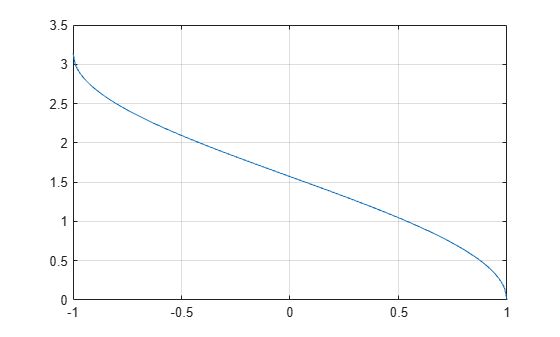# acos

## Syntax

``Y = acos(X)``

## Description

example

````Y = acos(X)` returns the Inverse Cosine (cos-1) of the elements of `X` in radians. The function accepts both real and complex inputs. For real values of `X` in the interval [-1, 1], `acos(X)` returns values in the interval [0, π].For real values of `X` outside the interval [-1,1] and for complex values of `X`, `acos(X)` returns complex values. ```

## Examples

collapse all

Find the inverse cosine of a value.

`y = acos(0)`
```y = 1.5708 ```

Find the inverse cosine of the elements of vector `x`. The `acos` function acts on `x` element-wise.

```x = [0.5i 1+3i -2.2+i]; y = acos(x)```
```y = 1×3 complex 1.5708 - 0.4812i 1.2632 - 1.8642i 2.6799 - 1.5480i ```

Plot the inverse cosine function over the intervals $-1\le x\le 1$.

```x = -1:.01:1; plot(x,acos(x)) grid on```## Input Arguments

collapse all

Cosine of angle, specified as a scalar, vector, matrix, or multidimensional array. The `acos` operation is element-wise when `X` is nonscalar.

Data Types: `single` | `double`
Complex Number Support: Yes

collapse all

### Inverse Cosine

The inverse cosine is defined as

`${\mathrm{cos}}^{-1}\left(z\right)=-i\mathrm{log}\left[z+i{\left(1-{z}^{2}\right)}^{1/2}\right].$`

## Version History

Introduced in R2006a